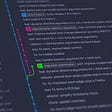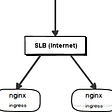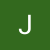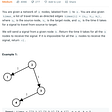# The Blind 75 Leetcode Series: Container With Most Water

Today, we will be working on Container with Most Water.

You are given an integer array `height` of length `n`. There are `n` vertical lines drawn such that the two endpoints of the `ith` line are `(i, 0)` and `(i, height[i])`.

Find two lines that together with the x-axis form a container, such that the container contains the most water.

Return the maximum amount of water a container can store.

Notice that you may not slant the container.

Compare to the previous questions we worked on, this one has a more detailed description, a welcoming cite. With a closer inspection of the problem, we can see that it’s giving us a list of numbers `height` with wants us to find the area between 2 lines.

What we can ask to enhance our understanding would be:

1. when we measure the area between 2 lines, do we simply ignore all lines in between?
2. Can height be 0?

If the answers are yes and yes, we can transform this problem to be

Given a list of numbers, find the max area between 2 numbers and their coordinates. Number can be 0 and treat the non-target lines as nonexistent.

The problem description is actually shorter now. With the visual aid, it still gives us a clear idea what we are looking for.

The first approach is always the brute force approach. We just try all the combinations of numbers and check for max.

`def maxArea(height):    n = len(height)    max_area = 0    for i in range(n):        for j in range(i, n):            current_area = (j - i) * min(height[i], height[j])            max_area = max(max_area, current_area)`

We get a new area each time the pointers move, and we compare that with the existing area. This covers the `height = 0` case as well.

What’s our time complexity in this case?

`def maxArea(height):    n = len(height)    max_area = 0    for i in range(n):  # O(n)        for j in range(i, n):  # O(n)            current_area = (j - i) * min(height[i], height[j])  # O(1)            max_area = max(max_area, current_area)  # O(1)`

We are looking at O(n²) for time complexity. Since this is the brute force approach, we should be able to do better.

An order of magnitude lower of O(n²) is O(n logn). This usually applies to some sort of binary search, which doesn’t apply here, so we go lower. A time complexity of O(n) means we can only go through the list once.

When we want to reduce the number of loops we go through, we need to track more components. In this case, we can have 2 pointers pointing to `i` and `j` we used in the brute force approach. Furthermore, we can start from the two ends and close in.

But how do we know which pointer to move in each iteration?

Since we are finding the max area, the intuition is that we want the maximum height and maximum width. We cannot control the width as `i` and `j` keep emerging, so we want to make sure we have the max height. Thus, if `height[i] <= height[j]`, we move `i`forwards. If `height[i] > height[j]`, we move `j` backwards.

If this doesn’t convince you, you can check chipbk10’s explanation and proof.

The iteration should end when `i` and `j` meet (since the area will be 0 at the point, and beyond that point, all calculations are already done. Ex. i=1, j=5 should get the same answer as i=5 and j=1).

With this approach, we will only traverse the list once, effectively making the time complexity reduce to O(n).

`def calculate_area(w, h):  # util function to make the code cleaner    return w * hdef maxArea(height):    n, max_area = len(height), 0    i, j = 0, n-1  # initialize the two pointers    while i < j:  # iteration ends when i meets j        max_area = max(calculateArea((j - i), min(height[i], height[j])), max_area)        if height[i] <= height[j]:  # move i if height[i] is smaller            i += 1        else:  # else move j            j -= 1            return max_area`

Looking at the time complexity again

`def calculate_area(w, h):  # O(1)    return w * hdef maxArea(height):    n, max_area = len(height), 0    i, j = 0, n-1    while i < j:  # we go through each element in the list, so O(n)        max_area = max(calculateArea((j - i), min(height[i], height[j])), max_area)  # O(1)        if height[i] <= height[j]:  # O(1)            i += 1        else:            j -= 1            return max_area`

And here we go, another problem down.

--

--

--

## More from Jonathan Chao

I am a software developer who has been in this industry for close to a decade. I share my experience to people who are or want to get into the industry

Love podcasts or audiobooks? Learn on the go with our new app.

## Create a PR to merge a fork’s changes using Github CLI## Databases — A System Design Perspective## How we created a cloud continuous integration service## Exporting Activity to System Settings## Configuring Ingress Controller of Container Service for Kubernetes to Use an Intranet SLB Instance## My road from a hillbilly to a professional developer## Securely Manage Secrets with HashiCorp Vault## Why is Service Mesh ?## Jonathan Chao

I am a software developer who has been in this industry for close to a decade. I share my experience to people who are or want to get into the industry

## The Blind 75 Leetcode Series: Spiral Matrix## Solutions for LeetCode 743## Coding Algorithms — Best Time To Buy and Sell Stocks